# Accessories

55 products found
Filtres

Gender

Size

Price

€0.00 - €70.00

Couleurs

Sport

Use• S
• M
• L
• XL• S
• M
• L
• XL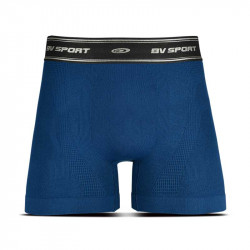• S
• M
• L
• XL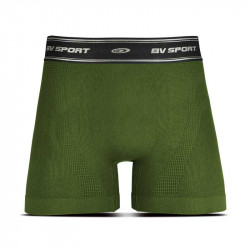• S
• M
• L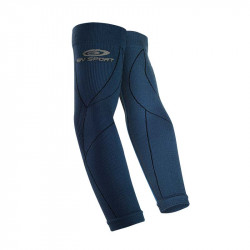• S/M• One Size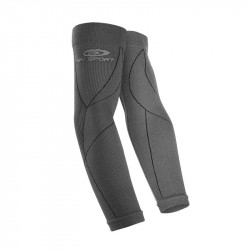• S/M
• L/XL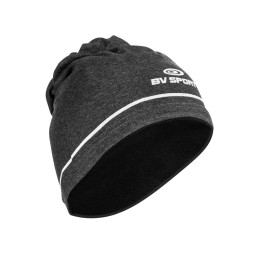• One Size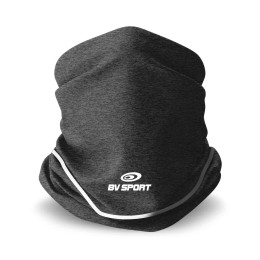• One Size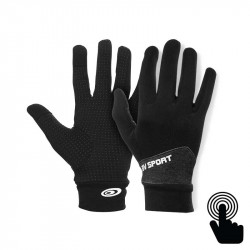• S/M
• L/XL• S/M
• L/XL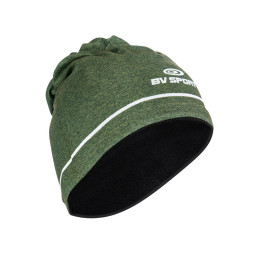• One Size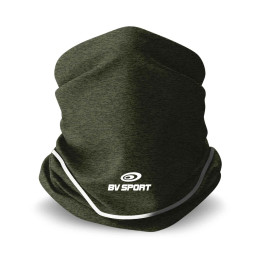• One Size• S/M
• L/XL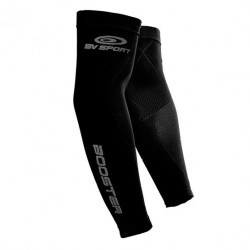• S/M
• L/XL• One Size• One Size• S/M
• L/XL• One Size• One Size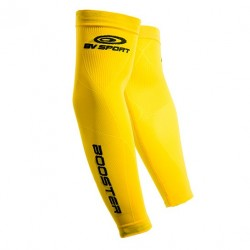• L/XL• S/M
• L/XL• One Size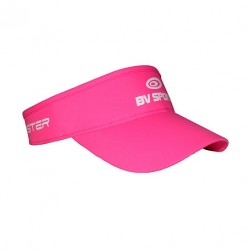-€10.00
• One Size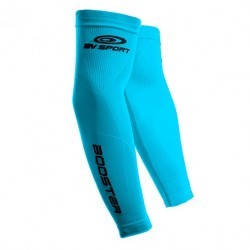• S/M
• L/XL• One Size-€10.00
• One Size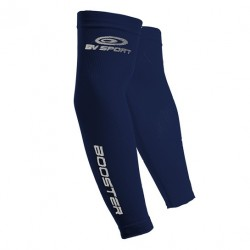• S/M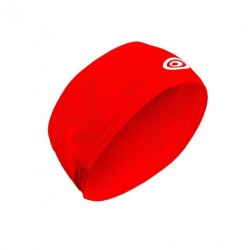• One Size-€10.00
• One Size• L/XL-€10.00
• One Size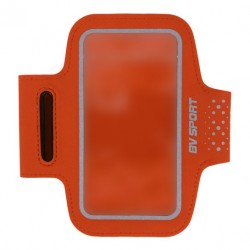-30%
• One Size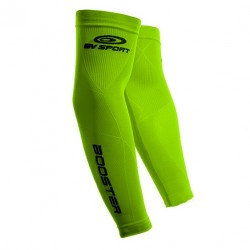• L/XL• S• One Size